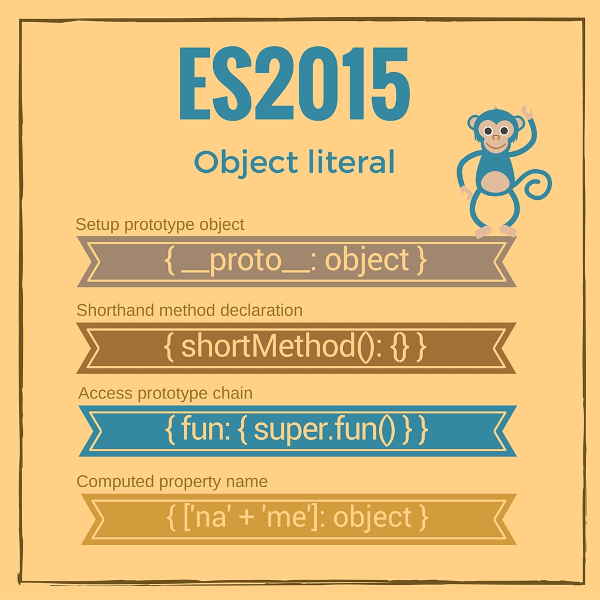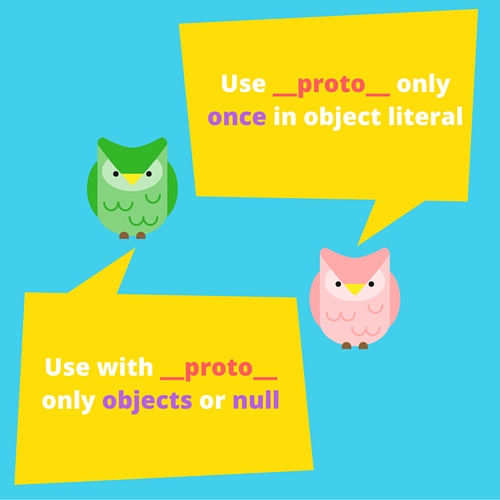08月03, 2016

# 【译】为什么我要说 JavaScript 对象字面量很酷？

ECMAScript 2015 之前，JavaScript 里的对象字面量（也叫对象初始化器）功能很弱。它只能定义两种属性：

• 普通键/值对 `{ name1: value }`
• Getters `{ get name(){..} }` 以及 setters `{ set name(val){..} }`，用来设置和获取需要计算的值。

Try in JS Bin

``````var myObject = {
myString: "value 1",
get myNumber() {
return this.myNumber;
},
set myNumber(value) {
this.myNumber = Number(value);
}
};
myObject.myString; // => "value 1"
myObject.myNumber = "15";
myObject.myNumber; // => 15
``````

JavaScript 是一个基于原型的语言，因此一切皆是对象。当涉及到对象创建、结构和访问原型时，语言必须提供简单的结构。

Try in JS Bin

``````var myProto = {
propertyExists: function(name) {
return name in this;
}
};
var myNumbers = Object.create(myProto);
myNumbers["array"] = [1, 6, 7];
myNumbers.propertyExists("array");      // => true
myNumbers.propertyExists("collection"); // => false
``````

• 在对象构造的过程中设置原型
• 速记方法定义
• 调用父类方法
• 计算属性名称### 1. 在对象构造时设置原型

Try in JS Bin

``````var myObject = {
name: "Hello World!"
};
myObject.__proto__;                         // => {}
myObject.__proto__.isPrototypeOf(myObject); // => true
``````

`myObject.__proto__` 返回 `myObject` 的原型对象。

Try in JS Bin

``````var myProto = {
propertyExists: function(name) {
return name in this;
}
};
var myNumbers = {
__proto__: myProto,
array: [1, 6, 7]
};
myNumbers.propertyExists("array");      // => true
myNumbers.propertyExists("collection"); // => false
``````

`myNumbers` 对象使用原型 `myProto` 创建，这可以通过特殊属性 `__proto__` 实现。

• 让它变得简单明了的过程是复杂的
• 让它变得复杂和难以理解却很容易

#### 2.1 使用 `__proto__` 的特例Try in JS Bin

``````var object = {
__proto__: {
toString: function() {
return "[object Numbers]"
}
},
numbers: [1, 5, 89],
__proto__: {
toString: function() {
return "[object ArrayOfNumbers]"
}
}
};
``````

JavaScript 限制了只允许使用 object 或者 `null` 作为 `__proto__` 属性的值。使用其它原生类型（如字符串、数值、布尔类型）或者 `undefined` 会被忽略，并不能改变对象的原型。

Try in JS Bin

``````var objUndefined = {
__proto__: undefined
};
Object.getPrototypeOf(objUndefined); // => {}
var objNumber = {
__proto__: 15
};
Object.getPrototypeOf(objNumber);    // => {}
``````

### 2. 速记方法定义

Try in JS Bin

``````var collection = {
items: [],
this.items.push(item);
},
get(index) {
return this.items[index];
}
};
collection.get(0); // => 15
``````

`add()``get()``collection` 中使用快捷的方式定义的方法。

### 3. 调用父类方法

Try in JS Bin

``````var calc = {
sumArray (items) {
return items.reduce(function(a, b) {
return a + b;
});
}
};
var numbers = {
__proto__: calc,
numbers: [4, 6, 7],
sumElements() {
return super.sumArray(this.numbers);
}
};
numbers.sumElements(); // => 17
``````

`calc``numbers` 对象的属性。在 `numbers` 的方法 `sumElements` 里面，要调用原型 `calc` 上的方法，可以使用 `super` 关键字： `super.sumArray()`

#### 3.1 使用 `super` 的限制

Try in JS Bin

``````var calc = {
sumArray (items) {
return items.reduce(function(a, b) {
return a + b;
});
}
};
var numbers = {
__proto__: calc,
numbers: [4, 6, 7],
sumElements: function() {
return super.sumArray(this.numbers);
}
};
// Throws SyntaxError: "super" keyword unexpected here
numbers.sumElements();
``````

### 4. 计算属性名称

Try in JS Bin

``````function prefix(prefStr, name) {
return prefStr + "_" + name;
}
var object = {};
object[prefix("number", "pi")] = 3.14;
object[prefix("bool", "false")] = false;
object; // => { number_pi: 3.14, bool_false: false }
``````

Try in JS Bin

``````function prefix(prefStr, name) {
return prefStr + "_" + name;
}
var object = {
[prefix("number", "pi")]: 3.14,
[prefix("bool", "false")]: false
};
object; // => { number_pi: 3.14, bool_false: false }
``````

`[prefix('number', 'pi')]` 通过计算 `prefix('number', 'pi')` 表达式来设置属性名称, 得到的结果是 `'number_pi'`

#### 4.1 `Symbol` 作为属性名

Symbols 也可以被用来计算属性名称，只需要将它包含在方括号中：`{ [Symbol('name')]: 'Prop value' }`

Try in JS Bin

``````var object = {
number1: 14,
number2: 15,
string1: "hello",
string2: "world",
[Symbol.iterator]: function *() {
var own = Object.getOwnPropertyNames(this),
prop;
while(prop = own.pop()) {
yield prop;
}
}
}
[...object]; // => ["number1", "number2", "string1", "string2"]
``````

`[Symbol.iterator]: function *() { }` 定义一个属性来用于迭代遍历对象自有属性。展开操作符 `[...object]` 使用迭代器并返回自有属性列表。

### 5. 展望未来：rest 和属性展开

Rest 属性 允许我们从解构赋值左侧使用对象来收集属性，看下面的例子：

Try in JS Bin

``````var object = {
propA: 1,
propB: 2,
propC: 3
};
let {propA, ...restObject} = object;
propA;      // => 1
restObject; // => { propB: 2, propC: 3 }
``````

Try in JS Bin

``````var source = {
propB: 2,
propC: 3
};
var object = {
propA: 1,
...source
}
object; // => { propA: 1, propB: 2, propC: 3 }
``````

### 6. 结论

JavaScript 在快速进步。

-- EOF --